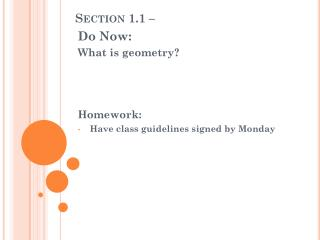DownloadDownload PresentationSection 1.1 –

# Section 1.1 –

Download Presentation## Section 1.1 –

- - - - - - - - - - - - - - - - - - - - - - - - - - - E N D - - - - - - - - - - - - - - - - - - - - - - - - - - -
##### Presentation Transcript

1. Section 1.1 – Do Now: What is geometry? Homework: Have class guidelines signed by Monday

2. Points, Lines, & Planes The three building blocks of geometry are: • Points • Lines • Planes

3. Collinear & Coplanar Collinear means on the same line. Example: Coplanar means on the same plane Example: E Points A, B, and C are collinear D F Points D, E, and F are coplanar

4. Collinear & Coplanar Practice Problem

5. Line segment Line Segment: Consists of two points called endpoints of the segment and all the point between them that are collinear with the two points. Example: How to Name It: AB or BA Line Segment Endpoints

6. Line Segment – Practice Problems

7. Congruent Segments Congruent Segments: Two segments that have the same measure or length Example: How to Name: C AC DC 3 cm 3 cm means “is to congruent to” A D

8. Congruent Segments

9. Midpoint • Bisects: Divides the segment into two congruent segments. • Midpointof a Segment: The point on the segment that is the same distance from both endpoints. Example: How to Name: R Q P R is the midpoint of

10. Midpoint – Practice Problems

11. Ray • Ray: Begins at a point and extends infinitely in one direction. Example: How to Name - Need two letters -First letter is the endpoint of the ray -Second letter is any other point the ray passes through R S

12. Ray – Practice Problems

13. Practice Problems From Textbook Pg. 33 3-6, 8-9, 11, 18, 22-25, 27

14. Closure • Write down three things that you learned today.